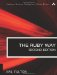# Section 5.13. Finding Prime Factorization, GCD, and LCM

### 5.12. Using mathn

For math-intensive programs, you will want to know about the excellent mathn library created by Keiju Ishitsuka. It provides a few convenience methods and classes, and in general helps to unify all of Ruby's numeric classes so that they "play well" together.

The easiest way to "use" this library is simply to require it and forget it. Because it requires the complex, rational, and matrix libraries (in that order), there is no need to do separate requires of those if you are using them.

In general, the mathn library tries to produce "sensible" results from computationsfor example, the square root of a Rational will be returned when possible as another Rational rather than a Float. Table 5.1 shows some typical effects of loading this library.

##### Table 5.1. Computation With and Without the mathn Library

Expression

Without mathn

With mathn

Math.sqrt(Rational(9,16))

0.75

Rational(3,4)

1/2

0

Rational(1,2)

Matrix.identity(3)/3

Matrix[[0,0,0], [0,0,0],[0,0,0]]

Matrix[[1/3,0,0], [0,1/3,0],[0,0,1/3]]

Math.sqrt(64/25)

1.4142...

Rational(8,5)

Rational(1,10).inspect

Rational(1,10)

1/10

The mathn library adds ** and power2 methods to Rational. It changes the behavior of Math.sqrt and adds the rational-aware function Math.rsqrt.

See also sections 5.13 "Finding Prime Factorization, GCD, and LCM" and 5.14, "Working with Prime Numbers."The Ruby Way, Second Edition: Solutions and Techniques in Ruby Programming (2nd Edition)
ISBN: 0672328844
EAN: 2147483647
Year: 2004
Pages: 269
Authors: Hal Fulton

Similar book on Amazon